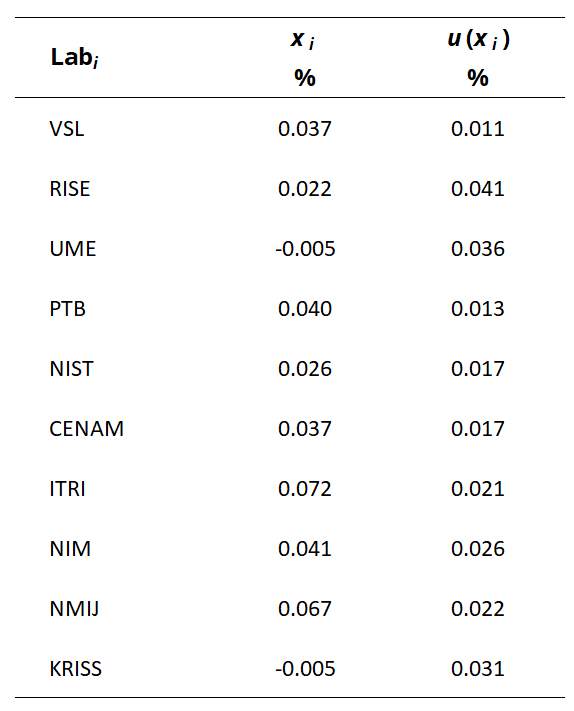Comparison
Comparison close
Results

CCM.FF-K1.2015

MEASURAND   Water flow

INSTRUMENT         Turbine meter

NOMINAL VALUE 30 m3/h

Degrees of equivalence represented by Di = (xI - xR) and its expanded uncertainty Ui at a 95 % level of confidence, both expressed in %.

Turbine meter 30 m3/h
Di / %
Participating institutes

CCM.FF-K1.2015

MEASURAND   Water flow

INSTRUMENT         Turbine meter

NOMINAL VALUE 60 m3/h

Degrees of equivalence represented by Di = (xI - xR) and its expanded uncertainty Ui at a 95 % level of confidence, both expressed in %.

Turbine meter 60 m3/h
Di / %
Participating institutes

CCM.FF-K1.2015

MEASURAND   Water flow

INSTRUMENT         Turbine meter

NOMINAL VALUE 100 m3/h

Degrees of equivalence represented by Di = (xI - xR) and its expanded uncertainty Ui at a 95 % level of confidence, both expressed in %.

Turbine meter 100 m3/h
Di / %
Participating institutes

CCM.FF-K1.2015

MEASURAND   Water flow

INSTRUMENT         Turbine meter

NOMINAL VALUE 140 m3/h

Degrees of equivalence represented by Di = (xI - xR) and its expanded uncertainty Ui at a 95 % level of confidence, both expressed in %.

Turbine meter 140 m3/h
Di / %
Participating institutes

CCM.FF-K1.2015

MEASURAND   Water flow

INSTRUMENT         Turbine meter

NOMINAL VALUE 180 m3/h

Degrees of equivalence represented by Di = (xI - xR) and its expanded uncertainty Ui at a 95 % level of confidence, both expressed in %.

Turbine meter 180 m3/h
Di / %
Participating institutes

CCM.FF-K1.2015

MEASURAND   Water flow

INSTRUMENT         Turbine meter

NOMINAL VALUE 200 m3/h

Degrees of equivalence represented by Di = (xI - xR) and its expanded uncertainty Ui at a 95 % level of confidence, both expressed in %.

Turbine meter 200 m3/h
Di / %
Participating institutes

CCM.FF-K1.2015

MEASURAND   Water flow

INSTRUMENT         Coriolis meter

NOMINAL VALUE 30 t/h

Degrees of equivalence represented by Di = (xI - xR) and its expanded uncertainty Ui at a 95 % level of confidence, both expressed in %.

Coriolis meter 30 t/h
Di / %
Participating institutes

CCM.FF-K1.2015

MEASURAND   Water flow

INSTRUMENT         Coriolis meter

NOMINAL VALUE 60 t/h

Degrees of equivalence represented by Di = (xI - xR) and its expanded uncertainty Ui at a 95 % level of confidence, both expressed in %.

Coriolis meter 60 t/h
Di / %
Participating institutes

CCM.FF-K1.2015

MEASURAND   Water flow

INSTRUMENT         Coriolis meter

NOMINAL VALUE 100 t/h

Degrees of equivalence represented by Di = (xI - xR) and its expanded uncertainty Ui at a 95 % level of confidence, both expressed in %.

Coriolis meter 100 t/h
Di / %
Participating institutes

CCM.FF-K1.2015

MEASURAND   Water flow

INSTRUMENT         Coriolis meter

NOMINAL VALUE 140 t/h

Degrees of equivalence represented by Di = (xI - xR) and its expanded uncertainty Ui at a 95 % level of confidence, both expressed in %.

Coriolis meter 140 t/h
Di / %
Participating institutes

CCM.FF-K1.2015

MEASURAND   Water flow

INSTRUMENT         Coriolis meter

NOMINAL VALUE 180 t/h

Degrees of equivalence represented by Di = (xI - xR) and its expanded uncertainty Ui at a 95 % level of confidence, both expressed in %.

Coriolis meter 180 t/h
Di / %
Participating institutes

CCM.FF-K1.2015

MEASURAND   Water flow

INSTRUMENT         Coriolis meter

NOMINAL VALUE 200 t/h

Degrees of equivalence represented by Di = (xI - xR) and its expanded uncertainty Ui at a 95 % level of confidence, both expressed in %.

Coriolis meter 200 t/h
Di / %
Participating institutes
Comparison
Comparison close
Results

CCM.FF-K1.2015

MEASURAND   Water flow

INSTRUMENT         Turbine meter

NOMINAL VALUE 30 m3/h

Degrees of equivalence represented by Di = (xI - xR) and its expanded uncertainty Ui at a 95 % level of confidence, both expressed in %.

LABi Di Uneg,i Upos,i
%
%
%
VSL 0.016 0.236
RISE -0.042 0.240
UME 0.055 0.244
PTB 0.050 0.233
CENAM -0.260 0.236
ITRI 0.158 0.235
NIM -0.011 0.237
NMIJ 0.030 0.236
KRISS 0.006 0.240
Participating institutes
CCM.FF-K1.2015

CCM.FF-K1.2015

MEASURAND   Water flow

INSTRUMENT         Turbine meter

NOMINAL VALUE 60 m3/h

Degrees of equivalence represented by Di = (xI - xR) and its expanded uncertainty Ui at a 95 % level of confidence, both expressed in %.

LABi Di Uneg,i Upos,i
%
%
%
VSL -0.012 0.204
RISE -0.068 0.207
UME 0.018 0.211
PTB 0.042 0.200
CENAM -0.043 0.202
ITRI 0.139 0.203
NIM -0.024 0.205
NMIJ -0.012 0.203
KRISS -0.046 0.208
Participating institutes
CCM.FF-K1.2015

CCM.FF-K1.2015

MEASURAND   Water flow

INSTRUMENT         Turbine meter

NOMINAL VALUE 100 m3/h

Degrees of equivalence represented by Di = (xI - xR) and its expanded uncertainty Ui at a 95 % level of confidence, both expressed in %.

LABi Di Uneg,i Upos,i
%
%
%
VSL -0.007 0.196
RISE -0.082 0.200
UME 0.009 0.205
PTB 0.026 0.192
CENAM -0.051 0.195
ITRI 0.162 0.195
NIM -0.013 0.197
NMIJ -0.004 0.196
KRISS -0.045 0.200
Participating institutes
CCM.FF-K1.2015

CCM.FF-K1.2015

MEASURAND   Water flow

INSTRUMENT         Turbine meter

NOMINAL VALUE 140 m3/h

Degrees of equivalence represented by Di = (xI - xR) and its expanded uncertainty Ui at a 95 % level of confidence, both expressed in %.

LABi Di Uneg,i Upos,i
%
%
%
VSL 0.026 0.192
RISE -0.081 0.196
UME -0.041 0.200
PTB 0.049 0.188
CENAM -0.073 0.191
ITRI 0.158 0.191
NIM -0.033 0.193
NMIJ 0.010 0.192
KRISS -0.025 0.196
Participating institutes
CCM.FF-K1.2015

CCM.FF-K1.2015

MEASURAND   Water flow

INSTRUMENT         Turbine meter

NOMINAL VALUE 180 m3/h

Degrees of equivalence represented by Di = (xI - xR) and its expanded uncertainty Ui at a 95 % level of confidence, both expressed in %.

LABi Di Uneg,i Upos,i
%
%
%
VSL 0.038 0.189
RISE -0.087 0.193
UME -0.055 0.198
PTB 0.048 0.185
CENAM -0.093 0.188
ITRI 0.175 0.188
NIM -0.041 0.191
NMIJ 0.016 0.189
KRISS -0.011 0.194
Participating institutes
CCM.FF-K1.2015

CCM.FF-K1.2015

MEASURAND   Water flow

INSTRUMENT         Turbine meter

NOMINAL VALUE 200 m3/h

Degrees of equivalence represented by Di = (xI - xR) and its expanded uncertainty Ui at a 95 % level of confidence, both expressed in %.

LABi Di Uneg,i Upos,i
%
%
%
VSL 0.045 0.191
RISE -0.090 0.195
UME -0.055 0.198
PTB 0.048 0.186
CENAM -0.108 0.189
ITRI 0.176 0.190
NIM -0.045 0.192
NMIJ 0.018 0.190
KRISS 0.000 0.195
Participating institutes
CCM.FF-K1.2015

CCM.FF-K1.2015

MEASURAND   Water flow

INSTRUMENT         Coriolis meter

NOMINAL VALUE 30 t/h

Degrees of equivalence represented by Di = (xI - xR) and its expanded uncertainty Ui at a 95 % level of confidence, both expressed in %.

LABi Di Uneg,i Upos,i
%
%
%
VSL -0.017 0.049
RISE -0.009 0.089
UME 0.020 0.082
PTB 0.004 0.044
NIST -0.028 0.051
CENAM 0.006 0.050
ITRI 0.028 0.056
NIM -0.018 0.064
NMIJ 0.032 0.059
KRISS -0.015 0.072
Participating institutes
CCM.FF-K1.2015

CCM.FF-K1.2015

MEASURAND   Water flow

INSTRUMENT         Coriolis meter

NOMINAL VALUE 60 t/h

Degrees of equivalence represented by Di = (xI - xR) and its expanded uncertainty Ui at a 95 % level of confidence, both expressed in %.

LABi Di Uneg,i Upos,i
%
%
%
VSL -0.013 0.025
RISE -0.009 0.083
UME 0.010 0.075
PTB 0.004 0.029
NIST -0.017 0.038
CENAM 0.012 0.036
ITRI 0.037 0.045
NIM -0.002 0.054
NMIJ 0.018 0.047
KRISS -0.040 0.064
Participating institutes
CCM.FF-K1.2015

CCM.FF-K1.2015

MEASURAND   Water flow

INSTRUMENT         Coriolis meter

NOMINAL VALUE 100 t/h

Degrees of equivalence represented by Di = (xI - xR) and its expanded uncertainty Ui at a 95 % level of confidence, both expressed in %.

LABi Di Uneg,i Upos,i
%
%
%
VSL -0.010 0.021
RISE -0.013 0.081
UME 0.006 0.072
PTB -0.001 0.025
NIST -0.020 0.037
CENAM 0.012 0.034
ITRI 0.045 0.043
NIM 0.004 0.052
NMIJ 0.025 0.045
KRISS -0.055 0.063
Participating institutes
CCM.FF-K1.2015

CCM.FF-K1.2015

MEASURAND   Water flow

INSTRUMENT         Coriolis meter

NOMINAL VALUE 140 t/h

Degrees of equivalence represented by Di = (xI - xR) and its expanded uncertainty Ui at a 95 % level of confidence, both expressed in %.

LABi Di Uneg,i Upos,i
%
%
%
VSL -0.002 0.020
RISE -0.011 0.081
UME -0.046 0.075
PTB 0.003 0.024
NIST -0.014 0.036
CENAM -0.001 0.033
ITRI 0.042 0.042
NIM 0.003 0.052
NMIJ 0.029 0.044
KRISS -0.064 0.061
Participating institutes
CCM.FF-K1.2015

CCM.FF-K1.2015

MEASURAND   Water flow

INSTRUMENT         Coriolis meter

NOMINAL VALUE 180 t/h

Degrees of equivalence represented by Di = (xI - xR) and its expanded uncertainty Ui at a 95 % level of confidence, both expressed in %.

LABi Di Uneg,i Upos,i
%
%
%
VSL -0.003 0.018
RISE -0.013 0.081
UME -0.022 0.072
PTB 0.000 0.022
NIST -0.033 0.044
CENAM 0.000 0.032
ITRI 0.050 0.041
NIM 0.002 0.051
NMIJ 0.031 0.043
KRISS -0.053 0.061
Participating institutes
CCM.FF-K1.2015

CCM.FF-K1.2015

MEASURAND   Water flow

INSTRUMENT         Coriolis meter

NOMINAL VALUE 200 t/h

Degrees of equivalence represented by Di = (xI - xR) and its expanded uncertainty Ui at a 95 % level of confidence, both expressed in %.

LABi Di Uneg,i Upos,i
%
%
%
VSL -0.001 0.018
RISE -0.016 0.081
UME -0.043 0.071
PTB 0.002 0.022
NIST -0.012 0.032
CENAM -0.001 0.032
ITRI 0.034 0.041
NIM 0.003 0.051
NMIJ 0.029 0.043
KRISS -0.043 0.061
Participating institutes
CCM.FF-K1.2015
Comparison
Comparison close
CC comparison
 Metrology area, Sub-field Mass and related quantities, Fluid Flow Description Water flow Time of measurements 2015 - 2017 Status Approved for equivalence
 Final Reports of the comparisons Measurand K-factor (pulses/L) and (pulses/kg) Water flow: 30 m3/h to 200 m3/h Parameters Test pipe diameter: 100 mm Transfer device Turbine flow meter, Coriolis flow meter
 Comparison type Key Comparison Consultative Committee CCM (Consultative Committee for Mass and Related Quantities) Conducted by CCM (Consultative Committee for Mass and Related Quantities) Pilot institute PTB Physikalisch-Technische Bundesanstalt Germany Contact person E. Frahm +49 531 592 1333
First Name Last Name
wwww@ww.www +356719836 Institute 1 Institute 1 Khmelnitskiy
Pilot laboratory
PTB

Physikalisch-Technische Bundesanstalt, Germany, EURAMET

 CENAM Centro Nacional de Metrologia, Mexico, SIM
 CMS/ITRI Industrial Technology Research Institute/Center for Measurement Standards, Chinese Taipei, APMP
 KRISS Korea Research Institute of Standards and Science, Korea, Republic of, APMP
 NIM National Institute of Metrology, China, APMP
 NIST National Institute of Standards and Technology, United States, SIM
 NMIJ AIST National Metrology Institute of Japan, Japan, APMP
 SP Technical Research Institute of Sweden from 2017 Research Institutes of Sweden AB, Sweden, EURAMET
 TUVNEL TUVNEL, United Kingdom, EURAMET
 UME TÜBITAK Ulusal Metroloji Enstitüsü, Türkiye, EURAMET
 VSL VSL, Netherlands, EURAMET
Comparison
Comparison close
Results

CCM.FF-K1.2015

MEASURAND   Water flow

INSTRUMENT         Turbine meter

NOMINAL VALUE 30 m3/h

The technique to determine key comparison reference value xR and its associated standard uncertainty uR is described in Section 5.3 of the CCM.FF-K1.2015 Final Report.

 Nominal value (m3/h) xR (%) uR (%) 30 0.263 0.042 60 0.422 0.036 100 0.502 0.035 140 0.585 0.034 180 0.688 0.034 200 0.727 0.034

The degree of equivalence of laboratory i relative to the key comparison reference value xR is given by a pair of terms, both expressed in %:

Di = (xi - xR) and its expanded uncertainty Ui at a 95 % level of confidence where Ui = 2 ui.

CCM.FF-K1.2015

MEASURAND   Water flow

INSTRUMENT         Turbine meter

NOMINAL VALUE 60 m3/h

The technique to determine key comparison reference value xR and its associated standard uncertainty uR is described in Section 5.3 of the CCM.FF-K1.2015 Final Report.

 Nominal value (m3/h) xR (%) uR (%) 30 0.263 0.042 60 0.422 0.036 100 0.502 0.035 140 0.585 0.034 180 0.688 0.034 200 0.727 0.034

The degree of equivalence of laboratory i relative to the key comparison reference value xR is given by a pair of terms, both expressed in %:

Di = (xi - xR) and its expanded uncertainty Ui at a 95 % level of confidence where Ui = 2 ui.

CCM.FF-K1.2015

MEASURAND   Water flow

INSTRUMENT         Turbine meter

NOMINAL VALUE 100 m3/h

The technique to determine key comparison reference value xR and its associated standard uncertainty uR is described in Section 5.3 of the CCM.FF-K1.2015 Final Report.

 Nominal value (m3/h) xR (%) uR (%) 30 0.263 0.042 60 0.422 0.036 100 0.502 0.035 140 0.585 0.034 180 0.688 0.034 200 0.727 0.034

The degree of equivalence of laboratory i relative to the key comparison reference value xR is given by a pair of terms, both expressed in %:

Di = (xi - xR) and its expanded uncertainty Ui at a 95 % level of confidence where Ui = 2 ui.

CCM.FF-K1.2015

MEASURAND   Water flow

INSTRUMENT         Turbine meter

NOMINAL VALUE 140 m3/h

The technique to determine key comparison reference value xR and its associated standard uncertainty uR is described in Section 5.3 of the CCM.FF-K1.2015 Final Report.

 Nominal value (m3/h) xR (%) uR (%) 30 0.263 0.042 60 0.422 0.036 100 0.502 0.035 140 0.585 0.034 180 0.688 0.034 200 0.727 0.034

The degree of equivalence of laboratory i relative to the key comparison reference value xR is given by a pair of terms, both expressed in %:

Di = (xi - xR) and its expanded uncertainty Ui at a 95 % level of confidence where Ui = 2 ui.

CCM.FF-K1.2015

MEASURAND   Water flow

INSTRUMENT         Turbine meter

NOMINAL VALUE 180 m3/h

The technique to determine key comparison reference value xR and its associated standard uncertainty uR is described in Section 5.3 of the CCM.FF-K1.2015 Final Report.

 Nominal value (m3/h) xR (%) uR (%) 30 0.263 0.042 60 0.422 0.036 100 0.502 0.035 140 0.585 0.034 180 0.688 0.034 200 0.727 0.034

The degree of equivalence of laboratory i relative to the key comparison reference value xR is given by a pair of terms, both expressed in %:

Di = (xi - xR) and its expanded uncertainty Ui at a 95 % level of confidence where Ui = 2 ui.

CCM.FF-K1.2015

MEASURAND   Water flow

INSTRUMENT         Turbine meter

NOMINAL VALUE 200 m3/h

The technique to determine key comparison reference value xR and its associated standard uncertainty uR is described in Section 5.3 of the CCM.FF-K1.2015 Final Report.

 Nominal value (m3/h) xR (%) uR (%) 30 0.263 0.042 60 0.422 0.036 100 0.502 0.035 140 0.585 0.034 180 0.688 0.034 200 0.727 0.034

The degree of equivalence of laboratory i relative to the key comparison reference value xR is given by a pair of terms, both expressed in %:

Di = (xi - xR) and its expanded uncertainty Ui at a 95 % level of confidence where Ui = 2 ui.

CCM.FF-K1.2015

MEASURAND   Water flow

INSTRUMENT         Coriolis meter

NOMINAL VALUE 30 t/h

The technique to determine key comparison reference value xR and its associated standard uncertainty uR is described in Section 5.3 of the CCM.FF-K1.2015 Final Report.

 Nominal value (t/h) xR (%) uR (%) 30 0.041 0.010 60 0.038 0.007 100 0.033 0.006 140 0.031 0.006 180 0.036 0.006 200 0.038 0.006

The degree of equivalence of laboratory i relative to the key comparison reference value xR is given by a pair of terms, both expressed in %:

Di = (xi - xR) and its expanded uncertainty Ui at a 95 % level of confidence where Ui = 2 ui.

CCM.FF-K1.2015

MEASURAND   Water flow

INSTRUMENT         Coriolis meter

NOMINAL VALUE 60 t/h

The technique to determine key comparison reference value xR and its associated standard uncertainty uR is described in Section 5.3 of the CCM.FF-K1.2015 Final Report.

 Nominal value (t/h) xR (%) uR (%) 30 0.041 0.010 60 0.038 0.007 100 0.033 0.006 140 0.031 0.006 180 0.036 0.006 200 0.038 0.006

The degree of equivalence of laboratory i relative to the key comparison reference value xR is given by a pair of terms, both expressed in %:

Di = (xi - xR) and its expanded uncertainty Ui at a 95 % level of confidence where Ui = 2 ui.

CCM.FF-K1.2015

MEASURAND   Water flow

INSTRUMENT         Coriolis meter

NOMINAL VALUE 100 t/h

The technique to determine key comparison reference value xR and its associated standard uncertainty uR is described in Section 5.3 of the CCM.FF-K1.2015 Final Report.

 Nominal value (t/h) xR (%) uR (%) 30 0.041 0.010 60 0.038 0.007 100 0.033 0.006 140 0.031 0.006 180 0.036 0.006 200 0.038 0.006

The degree of equivalence of laboratory i relative to the key comparison reference value xR is given by a pair of terms, both expressed in %:

Di = (xi - xR) and its expanded uncertainty Ui at a 95 % level of confidence where Ui = 2 ui.

CCM.FF-K1.2015

MEASURAND   Water flow

INSTRUMENT         Coriolis meter

NOMINAL VALUE 140 t/h

The technique to determine key comparison reference value xR and its associated standard uncertainty uR is described in Section 5.3 of the CCM.FF-K1.2015 Final Report.

 Nominal value (t/h) xR (%) uR (%) 30 0.041 0.010 60 0.038 0.007 100 0.033 0.006 140 0.031 0.006 180 0.036 0.006 200 0.038 0.006

The degree of equivalence of laboratory i relative to the key comparison reference value xR is given by a pair of terms, both expressed in %:

Di = (xi - xR) and its expanded uncertainty Ui at a 95 % level of confidence where Ui = 2 ui.

CCM.FF-K1.2015

MEASURAND   Water flow

INSTRUMENT         Coriolis meter

NOMINAL VALUE 180 t/h

The technique to determine key comparison reference value xR and its associated standard uncertainty uR is described in Section 5.3 of the CCM.FF-K1.2015 Final Report.

 Nominal value (t/h) xR (%) uR (%) 30 0.041 0.010 60 0.038 0.007 100 0.033 0.006 140 0.031 0.006 180 0.036 0.006 200 0.038 0.006

The degree of equivalence of laboratory i relative to the key comparison reference value xR is given by a pair of terms, both expressed in %:

Di = (xi - xR) and its expanded uncertainty Ui at a 95 % level of confidence where Ui = 2 ui.

CCM.FF-K1.2015

MEASURAND   Water flow

INSTRUMENT         Coriolis meter

NOMINAL VALUE 200 t/h

The technique to determine key comparison reference value xR and its associated standard uncertainty uR is described in Section 5.3 of the CCM.FF-K1.2015 Final Report.

 Nominal value (t/h) xR (%) uR (%) 30 0.041 0.010 60 0.038 0.007 100 0.033 0.006 140 0.031 0.006 180 0.036 0.006 200 0.038 0.006

The degree of equivalence of laboratory i relative to the key comparison reference value xR is given by a pair of terms, both expressed in %:

Di = (xi - xR) and its expanded uncertainty Ui at a 95 % level of confidence where Ui = 2 ui.

Comparison
Comparison close
Results

CCM.FF-K1.2015

MEASURAND   Water flow

INSTRUMENT         Turbine meter

NOMINAL VALUE 30 m3/h

xi   measurement value issued by laboratory iLabi

ui   combined standard uncertainty of xi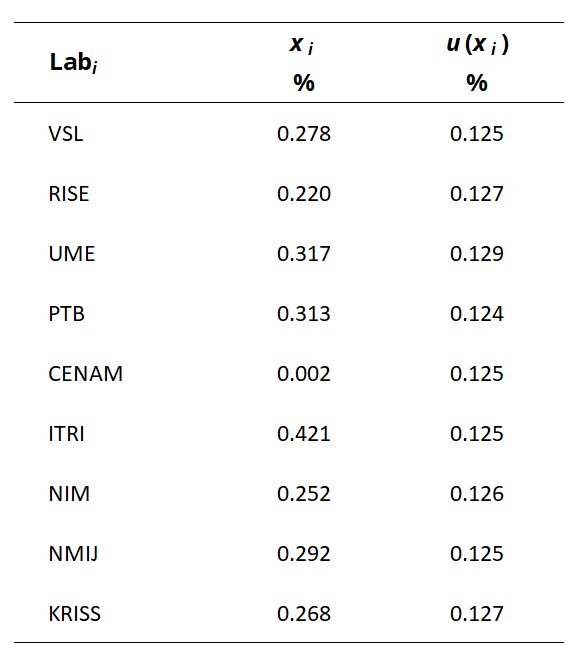CCM.FF-K1.2015

MEASURAND   Water flow

INSTRUMENT         Turbine meter

NOMINAL VALUE 60 m3/h

xi   measurement value issued by laboratory iLabi

ui   combined standard uncertainty of xi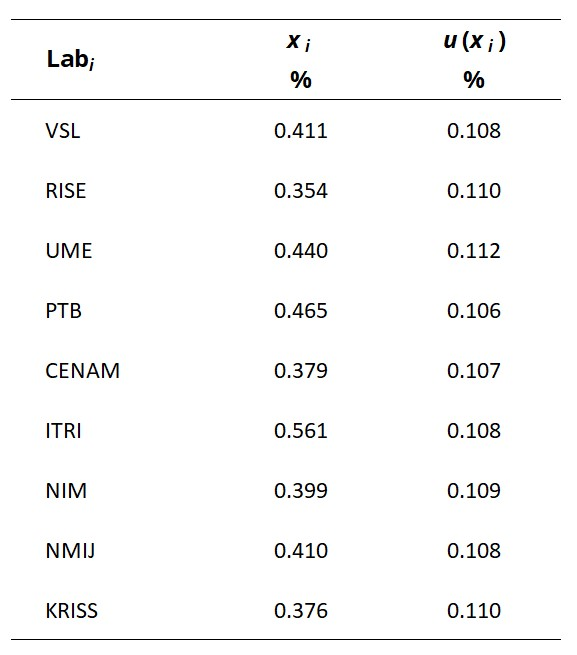CCM.FF-K1.2015

MEASURAND   Water flow

INSTRUMENT         Turbine meter

NOMINAL VALUE 100 m3/h

xi   measurement value issued by laboratory iLabi

ui   combined standard uncertainty of xi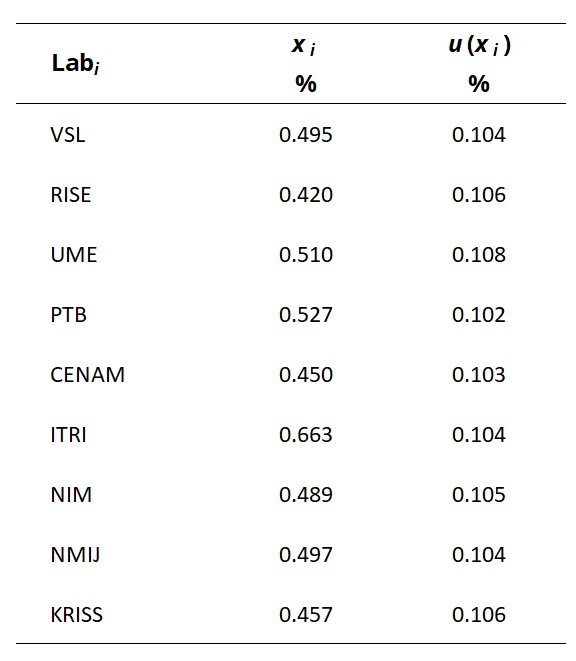CCM.FF-K1.2015

MEASURAND   Water flow

INSTRUMENT         Turbine meter

NOMINAL VALUE 140 m3/h

xi   measurement value issued by laboratory iLabi

ui   combined standard uncertainty of xi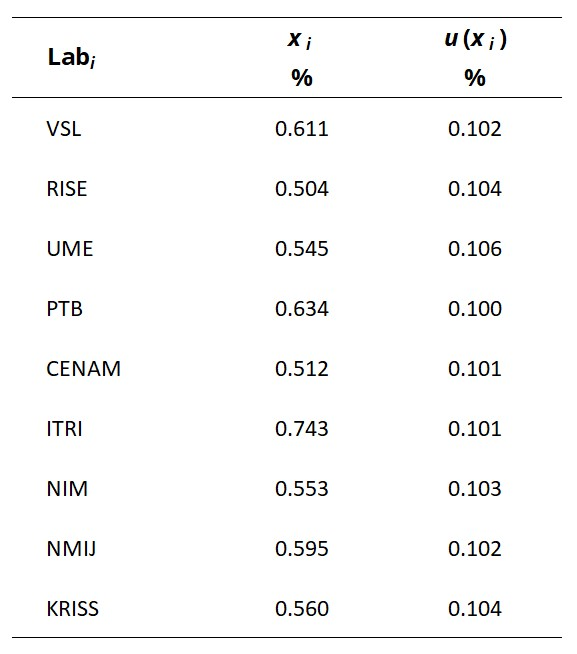CCM.FF-K1.2015

MEASURAND   Water flow

INSTRUMENT         Turbine meter

NOMINAL VALUE 180 m3/h

xi   measurement value issued by laboratory iLabi

ui   combined standard uncertainty of xi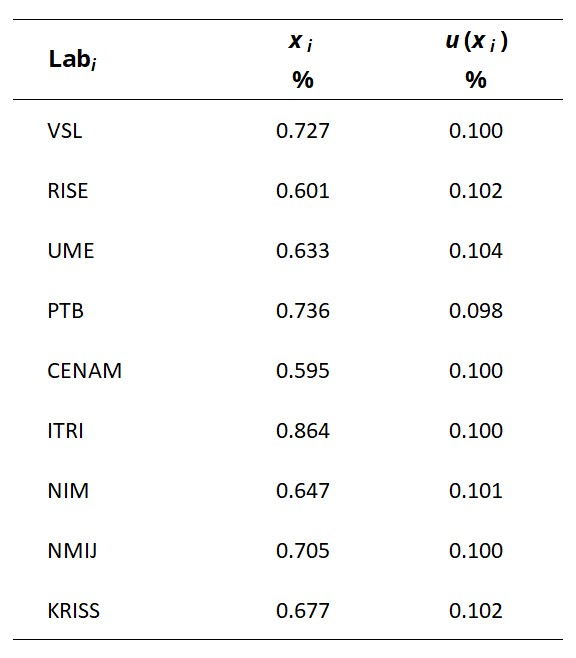CCM.FF-K1.2015

MEASURAND   Water flow

INSTRUMENT         Turbine meter

NOMINAL VALUE 200 m3/h

xi   measurement value issued by laboratory iLabi

ui   combined standard uncertainty of xi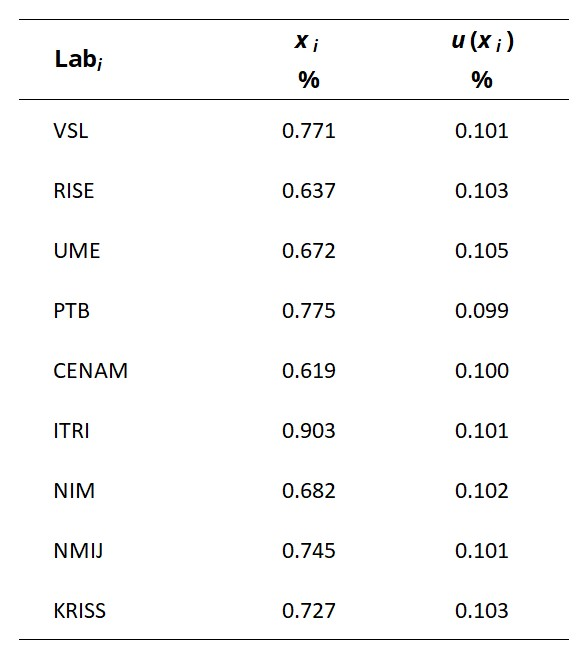CCM.FF-K1.2015

MEASURAND   Water flow

INSTRUMENT         Coriolis meter

NOMINAL VALUE 30 t/h

xi   measurement value issued by laboratory iLabi

ui   combined standard uncertainty of xi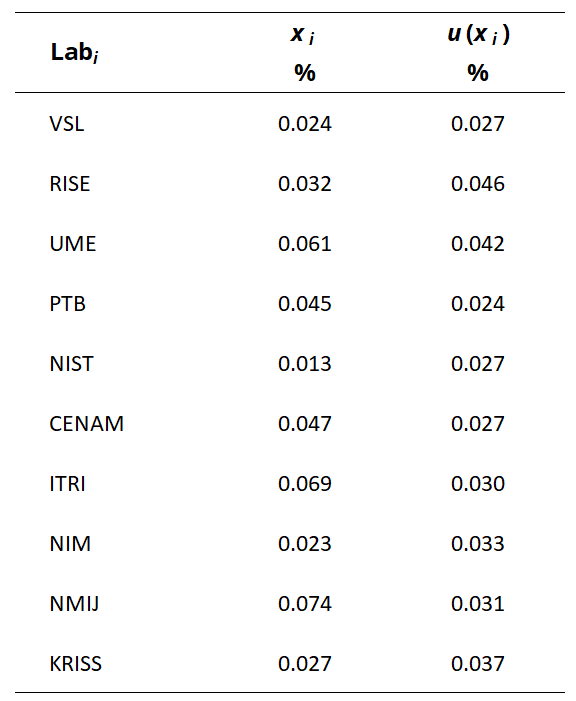CCM.FF-K1.2015

MEASURAND   Water flow

INSTRUMENT         Coriolis meter

NOMINAL VALUE 60 t/h

xi   measurement value issued by laboratory iLabi

ui   combined standard uncertainty of xi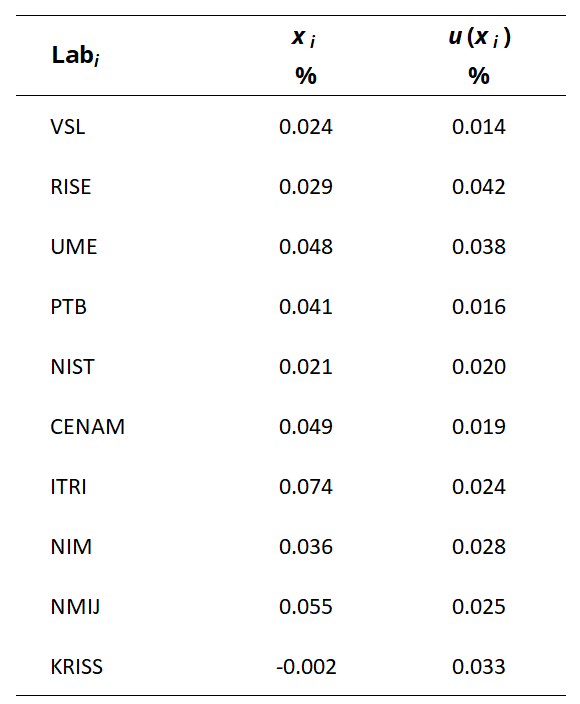CCM.FF-K1.2015

MEASURAND   Water flow

INSTRUMENT         Coriolis meter

NOMINAL VALUE 100 t/h

xi   measurement value issued by laboratory iLabi

ui   combined standard uncertainty of xi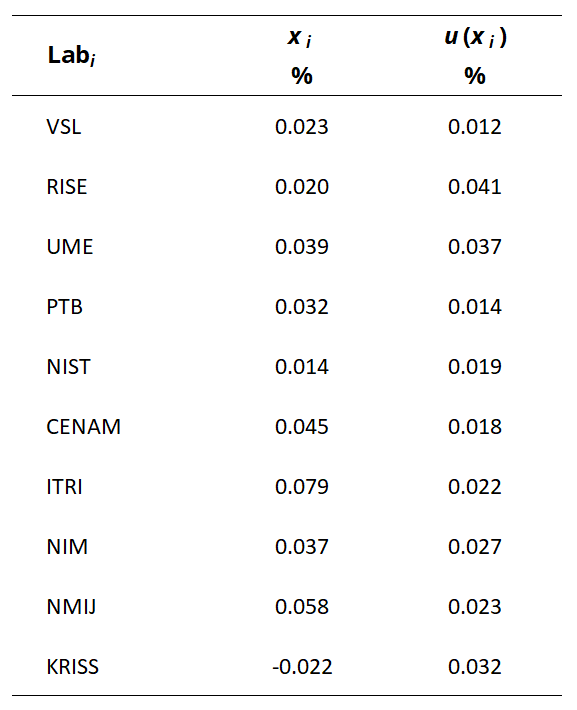CCM.FF-K1.2015

MEASURAND   Water flow

INSTRUMENT         Coriolis meter

NOMINAL VALUE 140 t/h

xi   measurement value issued by laboratory iLabi

ui   combined standard uncertainty of xiCCM.FF-K1.2015

MEASURAND   Water flow

INSTRUMENT         Coriolis meter

NOMINAL VALUE 180 t/h

xi   measurement value issued by laboratory iLabi

ui   combined standard uncertainty of xi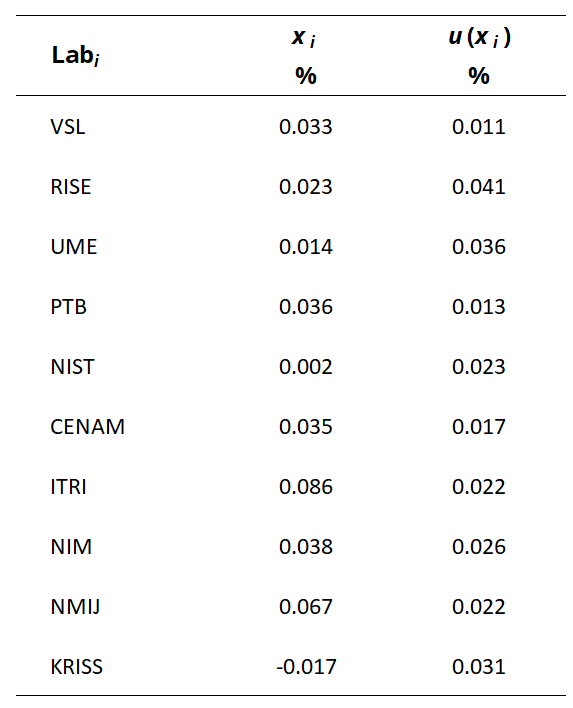CCM.FF-K1.2015

MEASURAND   Water flow

INSTRUMENT         Coriolis meter

NOMINAL VALUE 200 t/h

xi   measurement value issued by laboratory iLabi

ui   combined standard uncertainty of xi